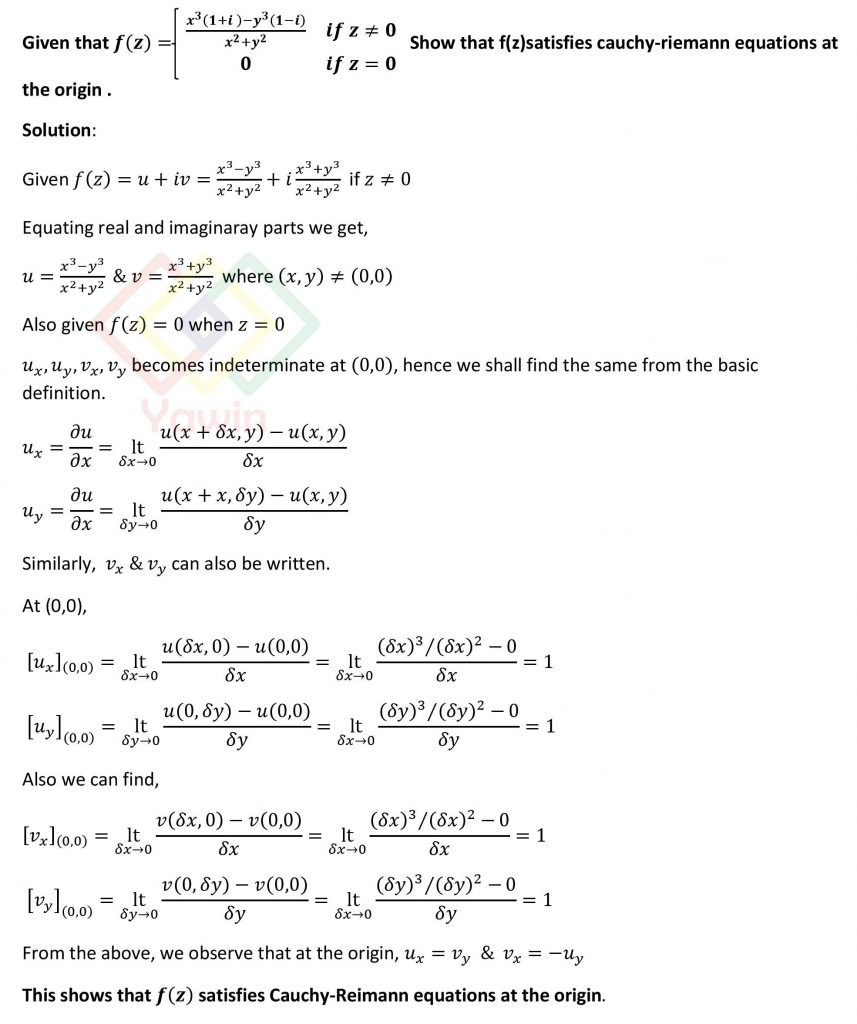Given that f(z)= (x^3 (1+i )-y^3 (1-i))/(x^2+y^2 ) if z≠0 and f(z) =0 if z=0, Show that f(z) satisfies Cauchy-Riemann equations at the origin

## Problem## Question

Given that f(z)= (x^3 (1+i )-y^3 (1-i))/(x^2+y^2 ) if z≠0 and f(z) =0 if z=0, Show that f(z) satisfies Cauchy-Riemann equations at the origin